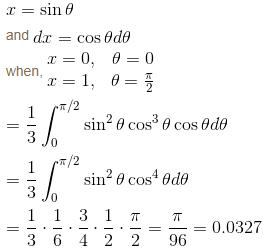Courses

# Integral Calculus NAT Level - 1

## 10 Questions MCQ Test Topic wise Tests for IIT JAM Physics | Integral Calculus NAT Level - 1

Description
This mock test of Integral Calculus NAT Level - 1 for Physics helps you for every Physics entrance exam. This contains 10 Multiple Choice Questions for Physics Integral Calculus NAT Level - 1 (mcq) to study with solutions a complete question bank. The solved questions answers in this Integral Calculus NAT Level - 1 quiz give you a good mix of easy questions and tough questions. Physics students definitely take this Integral Calculus NAT Level - 1 exercise for a better result in the exam. You can find other Integral Calculus NAT Level - 1 extra questions, long questions & short questions for Physics on EduRev as well by searching above.
*Answer can only contain numeric values
QUESTION: 1

### Find the area between the curves r = 3 cos θ and r = 2 - cos θ  is απ + β√3. Find the value of  α + β.

Solution: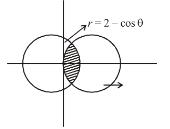The points of intersection are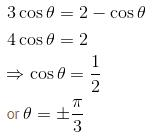Total Area = 2(Area of I + Area of II)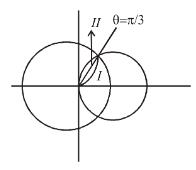Area of Region I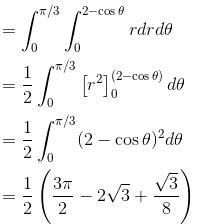Area of Region II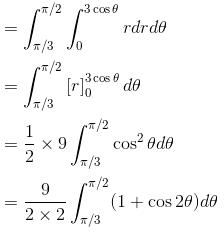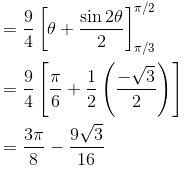Area of Region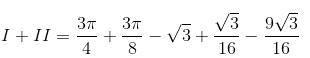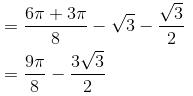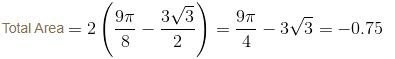*Answer can only contain numeric values
QUESTION: 2

### Find by Double Integration, whole area of the curve a2x2 = y3(2a – y). Let area be of the form λa2. Find value of λ.

Solution: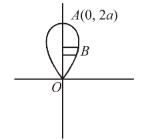As, it contains only even powers of x, hence, it is symmetrical about y-axis
Total Area = 2 × area OAB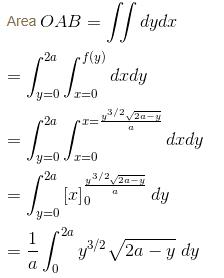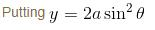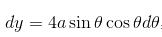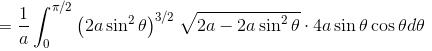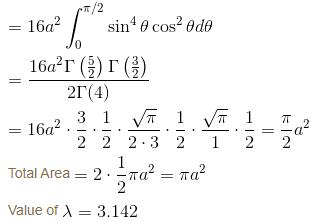*Answer can only contain numeric values
QUESTION: 3

### Find the whole area included between the curve x2y2 = a2(y2 – x2) and its asymptotes. It is of form λa2. Find value of λ.

Solution: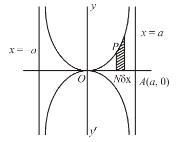The curve is
x2y2 = a2(y2 – x2)
(1) Symmetry about both the axes at even powers of x and y occur.
(2) Asymptotes are x = ±a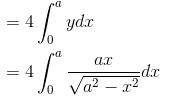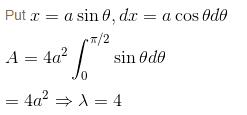*Answer can only contain numeric values
QUESTION: 4

Find the area bounded by the curve r = 4cos3θ. as given below :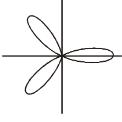Solution: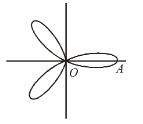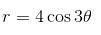Hence, the Area bounded is given as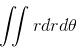As the curve is symmetrical.
Total Area = 3 × Area of loop OA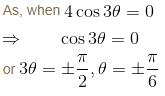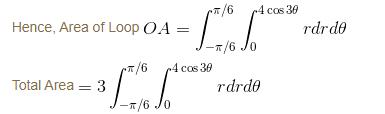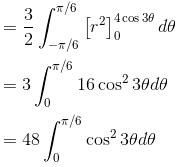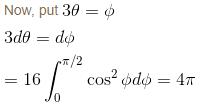= 12.571

*Answer can only contain numeric values
QUESTION: 5

If the area common to the circles r = a√2 and r = 2a cosθ is a2λ. Find the value of λ.

Solution:

Given curves are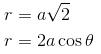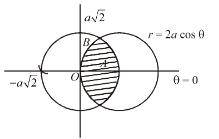Solving the two equations,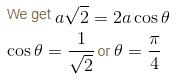Here Required area will be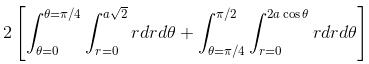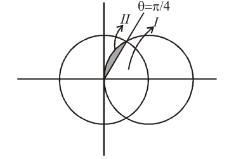For Region I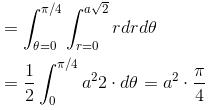For Region II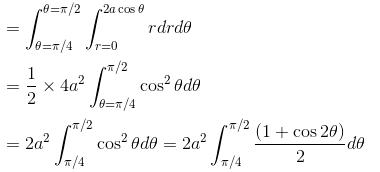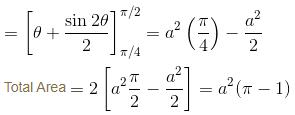Hence, λ = 2.142

*Answer can only contain numeric values
QUESTION: 6

The area bounded by the curve √x + √y = 1 and the coordinates axes is

Solution:

This can be done by two ways:
Ist Way: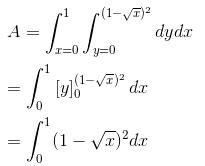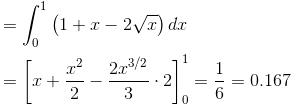IInd Way :
Putting x = 0 and y = 0, we find that the given curve meets y and x-axes in (0, 1) and (1, 0) respectively.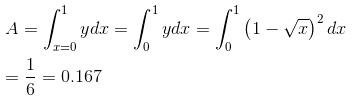*Answer can only contain numeric values
QUESTION: 7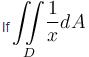where D is the region that lies inside the circle r = 3cosθ and outside the cadioid r = 1 + cos θ is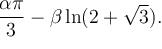Find the value of α . β.

Solution: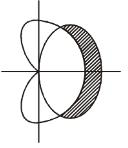Points of intersectionNotice that D is the region such that for each fixed angle θ between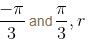varies from 1 + cos θ (cardioid) to 3 cos θ (circle).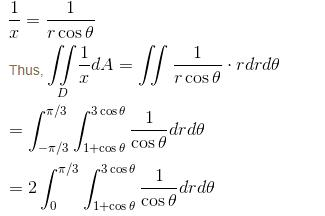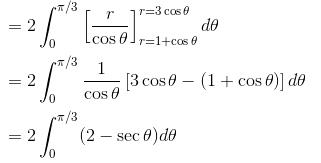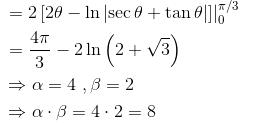*Answer can only contain numeric values
QUESTION: 8

Find the value of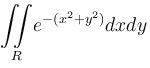where R is a circular disk x2 + y2 = a2 is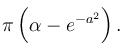Find the value of α.

Solution: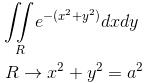Changing to polar coordinates, we get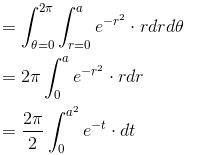Putting r2=t

2r dr = dt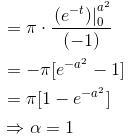*Answer can only contain numeric values
QUESTION: 9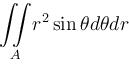over the area of cardioid r = a(1 + cosθ) above initial line is λa3.  Find the value of λ.

Solution: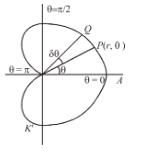The region of integration A can be covered by radial strips whose ends are at r = 0, r = a (1 + cos θ). The strips like between θ = 0 and θ = π.

Thus,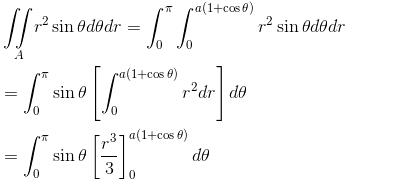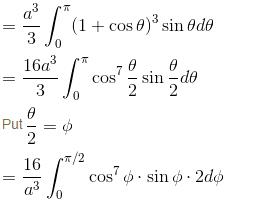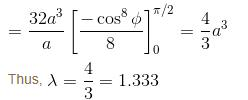*Answer can only contain numeric values
QUESTION: 10

Evaluate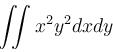over the domain {{x, y) : x > 0, y > 0, x2 + y2 < 1}

Solution: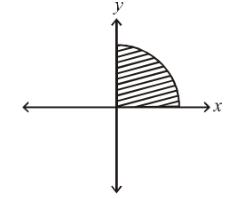The region of integration is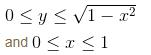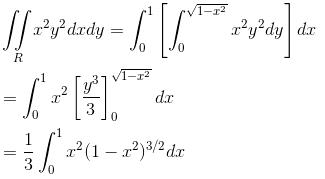Now, changing to polar coordinates, we get